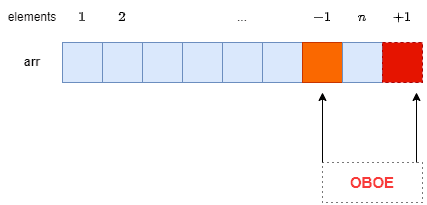## 1. Introduction

In this tutorial, we’ll study the off-by-one error (OBOE). This type of error is very common while iterating over a range of items.

We make this logical error when we either exceed the boundary by one or leave out the element before the boundary.

## 2. Concept

An off-by-one error is a type of error that is related to the start and end values of a contiguous range of memory.

In a typical scenario, we use a continuous block withmemory units to storeelements, each taking one unit. Let’s say we iterate over this blocktimes to read all the elements. But, if we access this block for eitherortimes, we have OBOE:## 3. Examples

Let’s go through different cases where OBOE can occur.

### 3.1. Different Indexing

In this scenario, we make this error when following a different index scheme than the underlying programming environment.

Let’s say the indices in the programming language start from 0. So, for an-element structure, the last index is. However, if we access it with an index ranging from 1 to, we get an OBOE.

We’ll use an example to clarify. Let’s say we have a 5-element array. Now, we iterate over it using indices 1 through 5. Here, we will get two errors, one of which is OBOE.

The first error will be that we will expectto return 2, whereas we’ll get 4.  The same goes forand. This is a logical error and is usually hard to catch.

The other error is a run-time OBOE that we get when trying to access the element. It’s outside the memory range allocated to, and hence the operating system will raise a memory error.

We can easily debug it by printing the current index in each iteration of the loop together with the upper and lower bounds of our memory structure. That way, we can check these two values to see which one is causing OBOE.

### 3.2. Looping Over a Sub-Array

This is very common when we don’t set the loop condition(s) properly.

Let’s say we have an arraywithelements and want to access. Further, the programming environment uses-based indexation. We’ll get an OBOE if we useas the last index to check:

``

Instead, we should use:

``

That way, we don’t miss the last element of the sub-array,.

## 4. Security Implications

OBOE has huge security implications when it overruns the memory range in our programs.

Let’s assume we allocate a block ofbytes on the heap. Then, we store a structurein it. Now, we try to updateby setting it to another structureof sizebytes. Here, we’ll corrupt a byte of the structure next toprovided that the programming language compiler (interpreter) doesn’t catch this error. In most cases, we’ll get a memory segmentation fault when this memory happens to belong to some other process.

Furthermore, on systems with little-endian architectures, this can overwrite the least significant byte of a memory structure. With this done, a hacker can place the starting address of a trojan or virus at this location. As a result, they can corrupt our system.

We can solve this problem by calculating the size of the new structurebefore the update operation. If the size ofis the same as that of, then only we update. Otherwise, we raise a size mismatch exception.

## 5. Solution

We can solve the off-by-one error in three ways.

The first is to dry-run every suspicious loop on paper before executing the code on a computer. That way, we can avoid any misjudgment about the index ranges.

The second way is to always specify the range of any contiguous structure as a half-open interval. For example, to select all theelements of an array, we use the range. It tells us to includebut not. Adopting a convention and sticking to it will greatly reduce the chance of getting an OBOE.

The third way is to include all boundary cases in our unit test cases. This way, we can check the code for all ranges. A unit test is an automated test script that we use to ensure that a stand-alone section of our code (we call it a unit) behaves as per its design and specification.

## 6. Conclusion

In this article, we explained the concept of the off-by-one error. It’s a logical error we make when we process one element more or less than we intended to.

This error is hard to catch. Thus, we need to be careful when specifying looping conditions.